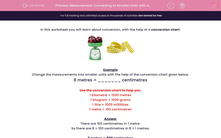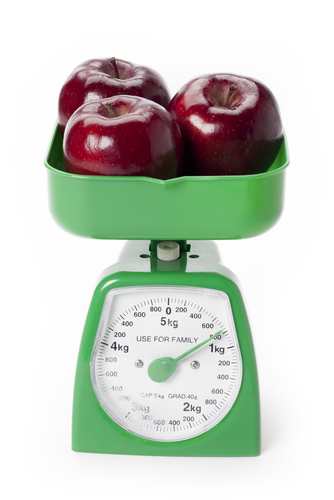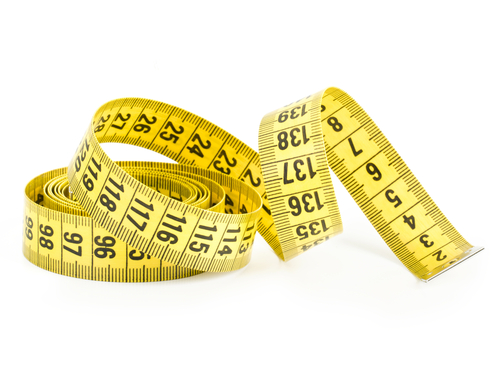# Measurement: Converting to Smaller Units with a Chart

In this worksheet, students convert metric measurements into smaller units using a conversion chart.This content is premium and exclusive to EdPlace subscribers.Key stage:  KS 2

Curriculum topic:   Maths and Numerical Reasoning

Difficulty level:#### Worksheet Overview

In this worksheet you will learn about conversion, with the help of a conversion chart.Example

Change the measurements into smaller units with the help of the conversion chart given below.

8 metres = _______ centimetres

1 kilometre = 1000 metres

1 kilogram = 1000 grams

1 litre = 1000 millilitres

1 metre = 100 centimetres

There are 100 centimetres in 1 metre.

So there are 8 × 100 centimetres in 8 × 1 metres.

8 metres = 800 centimetres

### What is EdPlace?

We're your National Curriculum aligned online education content provider helping each child succeed in English, maths and science from year 1 to GCSE. With an EdPlace account you’ll be able to track and measure progress, helping each child achieve their best. We build confidence and attainment by personalising each child’s learning at a level that suits them.

Get started